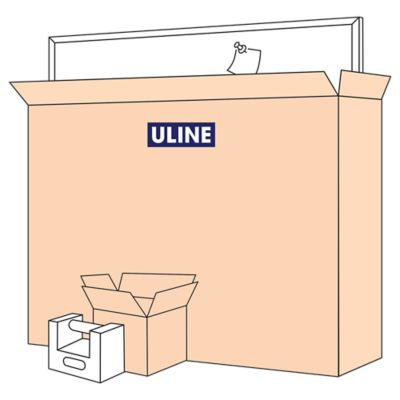# What is UPS dimensional (DIM) weight?

Dimensional (DIM) weight, also known as volumetric weight, is a calculation created by carriers such as UPS to apply rates based on a parcel's length, width and height. Dimensional weight takes into account package density to determine shipping rates.

How is dimensional weight calculated?

To calculate dimensional (DIM) weight, multiply the length, width and height of a package, using the longest point on each side. Then, divide the cubic size of the package in inches by the DIM divisor to calculate the dimensional weight in pounds.

The DIM divisor is a set number determined by each carrier. Please check the UPS website or your carrier for information pertaining to the latest DIM divisor.

Cast Iron Calibration Weight
Actual weight 50 lbs.
Dimensional weight (8 L x 6 W x 10" H) / DIM Divisor

Corkboard with Aluminum Frame
Actual weight 22 lbs.
Dimensional weight (60 L x 4 W x 36" H) / DIM Divisor

How to Calculate Dimensional WeightL x W x H

UPS Dim Divisor

Over 1,700 quality products in stock!

02/08/2023 07:51:02 AM; USWEB10 -0-0/0.0-1- 00000000-0000-0000-0000-000000000000